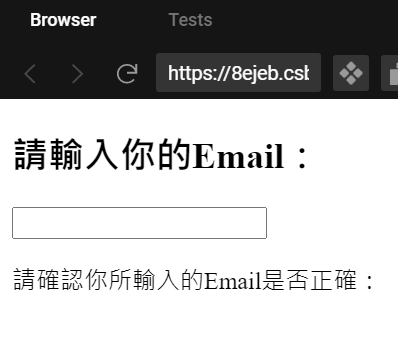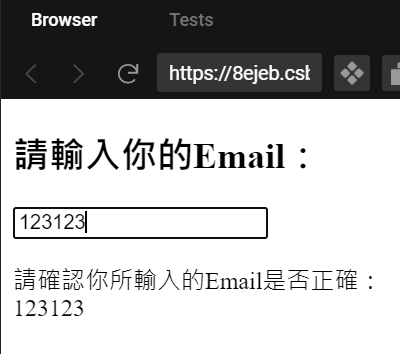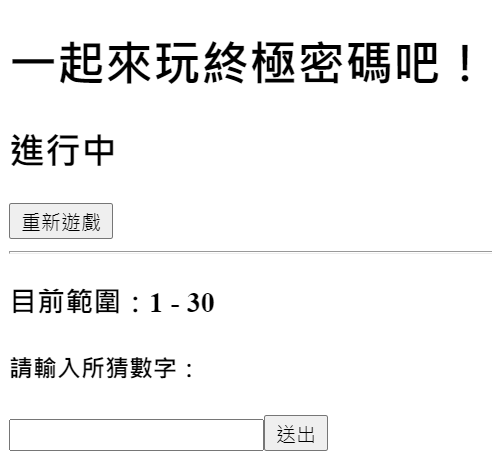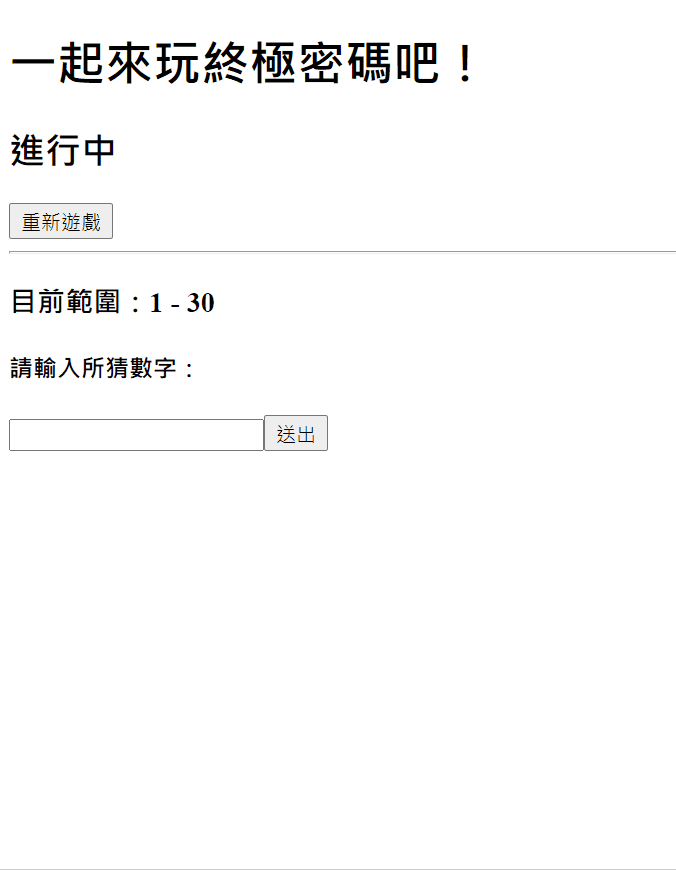#DAY 26
0

## 本日正文

### useState + `<input>`

``````<input type="string" value={email} />
``````

``````const [email, setEmail] = useState('');
``````

``````return (
<>
<h2>請輸入你的Email：</h2>
<input type="string" value={email} />
<p>
請確認你所輸入的Email是否正確：
<br />
{email}
</p>

</>
);
````````````const handleChange = (event) => {
setEmail(event.target.value);
}
``````

``````<input type="string" value={email} onChange={handleChange} />
``````### 終極密碼 - 前置作業

(PS. 如果有對終極密碼規則不了解的可以看維基介紹→ 終極密碼 )

1. 正確答案數字
2. 輸入的數字
3. 目前數字範圍

``````const [answer, setAnswer] = useState(17);
const [inputNum, setInputNum] = useState();
const [guessRange, setGuessRange] = useState([1,30]);
``````

``````if (event.target.value !== "") {
setInputNum(parseInt(event.target.value, 10));
} else {
setInputNum("");
}
``````

``````return (
<>
<h3>目前範圍：{guessRange} - {guessRange}</h3>
<h4>請輸入所猜數字：{inputNum}</h4>
<input type="number" value={inputNum} onChange={handleInput} />
<button>送出</button>
</>
)
``````### 終極密碼 - 正篇

``````const handleChange = (event) => {
if (
inputNum !== "" &&
inputNum > guessRange &&
inputNum < guessRange
) {
... (略)
}
}
};
``````

``````...(略)
if (
inputNum !== "" &&
inputNum > guessRange &&
inputNum < guessRange
) {
setGuessRange([guessRange, inputNum]);
setInputNum("");
return;
}
setGuessRange([inputNum, guessRange]);
setInputNum("");
return;
}
setStatus("恭喜答對！");
setInputNum("");
return;
}
}
``````

1. 如果輸入數字比正確答案大，
表示新的數字範圍將會落在 起始值~輸入數字
2. 如果輸入數字比正確答案小，
表示新的數字範圍將會落在 輸入數字~結尾值
3. 如果輸入數字跟正確答案一樣，
這就不用多說就是答對了XD
(PS. 我目前還沒做如果範圍被逼進到中間只夾正確答案要算答對的事XD)

``````<button onClick={handleChange}>送出</button>
``````

### 終極密碼 - 番外篇

``````const randomNum = Math.floor(Math.random() * 30 + 1);
``````

(PS. 一開始我先把答案寫死，寫完確定邏輯正確後我才把數字改成隨機產生)

``````const resetState = () => {
setInputNum("");
setGuessRange([1, 30]);
setStatus("進行中");
};
<button onClick={resetState}>重新遊戲</button>
``````### 終極密碼 - 成果篇